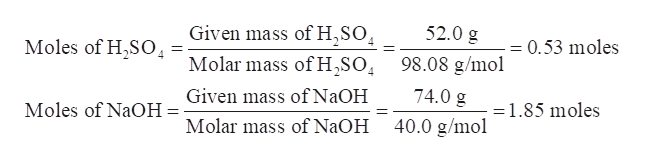Question
3 views
check_circle

Step 1

Aqueous sulfuric acid (H2SO4) reacts with solid NaOH to produce aqueous sodium sulfate (Na2SO4) and liquid water (H2O). If 52.0 g of sulfuric acid is mixed with 74.0 g of sodium hydroxide, then the maximum mass of sodium sulfate produced is to be calculated.

Step 2

Write the balanced chemical equation for the given reaction of sulfuric acid with sodium hydroxide.

Sulfuric acid + sodium hydroxide →Sodium sulfate + water

Step 3

Determine the given moles of H2SO4 and NaOH-

Given- mass of H2SO4 =52.0 g

Molar mass of H2SO4 = 98.08 g/mol

and

Mass of NaOH= ...help_outlineImage TranscriptioncloseGiven mass of H,SO Molar mass of H2SO 52.0 g Moles of H2SO -= 0.53 moles 98.08 g/mol Given mass of N2OH 74.0 g Moles of NaOH =1.85 moles 40.0 g/mo Molar mass of N2OH fullscreen

### Want to see the full answer?

See Solution

#### Want to see this answer and more?

Solutions are written by subject experts who are available 24/7. Questions are typically answered within 1 hour.*

See Solution
*Response times may vary by subject and question.
Tagged in

### General Chemistry